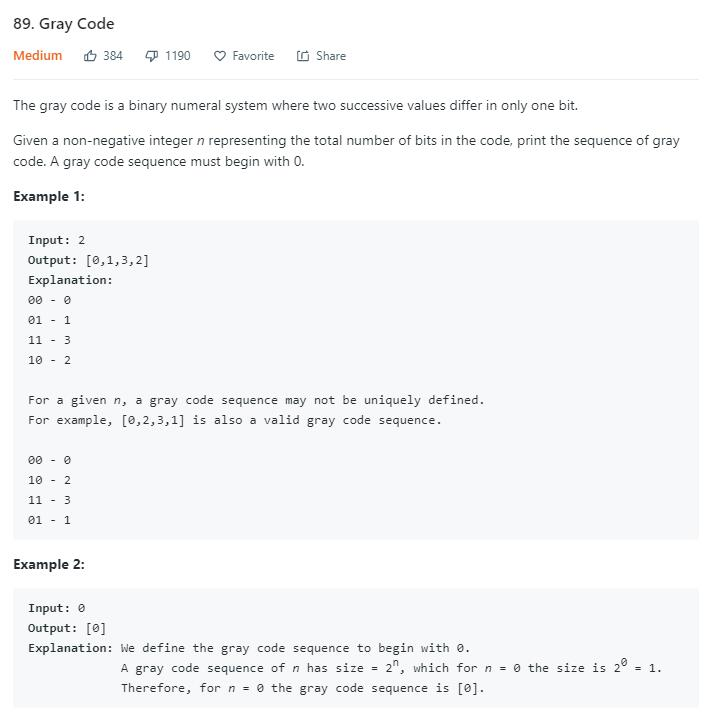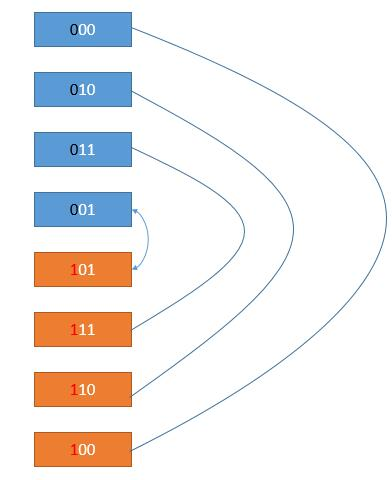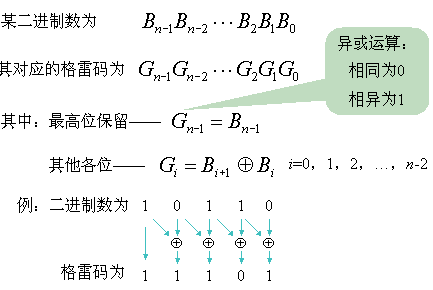# 题目描述（中等难度）# 解法一 动态规划

n = 2 的解
00 - 0
10 - 2
11 - 3
01 - 1


n = 3 的解，最高位是 0
000 - 0
010 - 2
011 - 3
001 - 1


n = 3 的解
000 - 0
010 - 2
011 - 3
001 - 1
------------- 下面的是新增的
100 - 4
110 - 6
111 - 7
101 - 5public List<Integer> grayCode(int n) {
List<Integer> gray = new ArrayList<Integer>();
gray.add(0); //初始化 n = 0 的解
for (int i = 0; i < n; i++) {
int add = 1 << i; //要加的数
//倒序遍历，并且加上一个值添加到结果中
for (int j = gray.size() - 1; j >= 0; j--) {
}
}
return gray;
}


# 解法二 直接推导

0 0 0 第零项初始化为 0。

0 0 1 第一项改变上一项最右边的位元

0 1 1 第二项改变上一项右起第一个为 1 的位元的左边位

0 1 0 第三项同第一项，改变上一项最右边的位元

1 1 0 第四项同第二项，改变最上一项右起第一个为 1 的位元的左边位

1 1 1 第五项同第一项，改变上一项最右边的位元

1 0 1 第六项同第二项，改变最上一项右起第一个为 1 的位元的左边位

1 0 0 第七项同第一项，改变上一项最右边的位元

public List<Integer> grayCode2(int n) {
List<Integer> gray = new ArrayList<Integer>();
for (int i = 1; i < 1 << n; i++) {
//得到上一个的值
int previous = gray.get(i - 1);
//同第一项的情况
if (i % 2 == 1) {
previous ^= 1; //和 0000001 做异或，使得最右边一位取反
//同第二项的情况
} else {
int temp = previous;
//寻找右边起第第一个为 1 的位元
for (int j = 0; j < n; j++) {
if ((temp & 1) == 1) {
//和 00001000000 类似这样的数做异或，使得相应位取反
previous = previous ^ (1 << (j + 1));
break;
}
temp = temp >> 1;
}
}
}
return gray;
}


# 解法三 公式public List<Integer> grayCode(int n) {
List<Integer> gray = new ArrayList<Integer>();
for(int binary = 0;binary < 1 << n; binary++){
}
return gray;
}